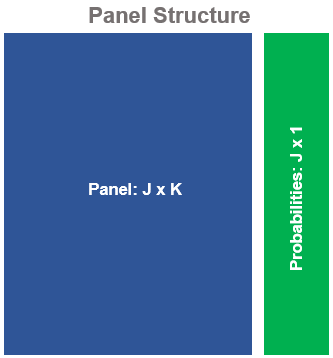# How does EP work?

Entropy-pooling (EP) is a powerful Bayesian technique that can be used to construct and process views on many elements of a multivariate distribution. Entropy-pooling enhances the Black-Litterman (1990)1 model by supporting views on non-normal markets, non-linear payoffs, tails of the distribution and more. As a result, the portfolio construction process can be substantially enriched.

Formally, EP relies on the Kullback-Leibner divergence to quantify the entropy between different distributions. The main goal is to minimize:

$\sum_{j=1}^{J} {x_j}(ln(x_j) - ln(p_j))$ Subject to the restrictions:

$Fx_j \le f \\ Hx_j = h$

In which $$x_j$$ is a yet to be discovered posterior probability; $$p_j$$ is a prior probability distribution; and the vectors $$F$$, $$f$$, $$H$$ and $$h$$ (i.e. the views) act as linear constraints on $$x_j$$.

When $$x_j = p_j$$, the relative entropy is zero and the two distributions coincide (i.e. the user views are in complete agreement with the market historical distribution). This is often unrealistic. Most commonly, the views will differ and the objective function will seek a value for $$x_j$$ that deviates from $$p_j$$ with the minimal possible adjustment, just the enough to incorporate the views.

The Lagrange can be formulated as:

$\mathcal{L}(x, \lambda, v) = x'(ln(x) - ln(p)) + \lambda'(Fx - f) + \nu'(Hx - h)$ To which $$\lambda$$ is the Lagrange multiplier for the inequality constraint and $$\nu$$ is the multiplier for the equality constraint. The subscript $$j$$ is drooped to light the notation.

The first order condition with respect to $$x$$ yields:

$\frac{\partial \mathcal{L}}{\partial x} = ln(x) - ln(p) + 1 + F'\lambda + H'\nu$ Set $$\frac{\partial \mathcal{L}}{\partial x} = 0$$ and separate the elements that contain $$x$$ from the rest to get:

$ln(x) = ln(p) - 1 - F'\lambda - H'\nu$ As a last step, exponentiate both sides to find a closed form solution for $$x$$:

$x(\lambda, v) = e^{ln(p) - 1 - F'\lambda - H'\nu}$

The solution is always positive and $$x \ge 0$$ is always satisfied. Nevertheless, $$x$$ still depends on the parameters, $$\lambda$$ and $$\nu$$, that can take any value.

In order to solve for $$\lambda$$ and $$\nu$$, set the dual formulation:

$D(\lambda, \nu) = \mathcal{L}(x(\lambda, \nu), \lambda, \nu) \\ s.t. \ \lambda \ge 0, \ \nu \$

This expression can be solved numerically, which allows to recover $$\lambda^*$$ and $$\nu^*$$, the optimal values for the Lagrange multipliers.

Plug $$\lambda^*$$ and $$\nu^*$$ in the optimal expression for $$x(\lambda, \nu)$$:

\begin{align*} x(\lambda, \nu) &= e^{ln(p) - 1 - F'\lambda - H'\nu} \\ x^*(\lambda^*, \nu^*) &= e^{ln(p) - 1 - F'\lambda^* - H'\nu^*} \\ x^*(\lambda^*, \nu^*) &= p^* \end{align*}

To get the probability vector that incorporates the views by distorting the “least” the original probability vector.

Notice that the dual optimization only works on the multipliers $$\lambda$$ and $$\nu$$ (the number of constraints in the original problem). This “dimensionality reduction” is exactly what makes entropy-pooling feasible, even when the number of scenarios in the original dataset is large.

In other words, for every $$J$$ realization (historical or simulated) the computational complexity is shrinked, because entropy-pooling only prices the probabilities of each scenario, not the scenarios themselves (the $$J \times K$$ panel is treated as fixed).Since every realization in the $$J \times K$$ panel is connected to the $$J \times 1$$ vector of posterior probabilities, the conditional statistics on the P&L can be computed super fast.2. As a result, EP can be used on “real-time”, without the computational burden of traditional Bayesian techniques.

1. Black, Fisher and Letterman, Robert (1990), Global Portfolio Optimization, Financial Analyst Journal, September 1992.↩︎

2. See the functions ffp_moments() and empirical_stats().↩︎